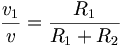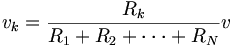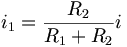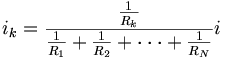# Voltage and Current Dividers

## Voltage Division

When we have a voltage across a string of resistors connected in series, the voltage across the entire string will be divided up among the resistors. We can express the voltage across a single resistor as a ratio of voltages and resistances, without ever knowing the current.

In the circuit above,$\frac{v_1}{v}=\frac{R_1}{R_1+R_2}$

or$v_1=\frac{R_1}{R_1+R_2}v$

We can generalize this equation for N resistors in series with the equation:$v_k=\frac{R_k}{R_1+R_2+\cdot\cdot\cdot+R_N}v$

where vk is the voltage across resistor k and v is the voltage across the whole string of resistors.

## Current Division

Resistors in parallel divide up the current. When we have a current flowing through resistors in parallel, we can express the current flowing through a single resistor as ratio of currents and resistances, without ever knowing the voltage.

In the circuit above$\frac{i_1}{i}=\frac{R_2}{R_1+R_2}$

or$i_1=\frac{R_2}{R_1+R_2}i$

where i is the current flowing through all the resistors. Note that the numerator on the right is R2, not R1. Remember that a larger resistance will carry a smaller current.

We can generalize the equation for N resistors in parallel with the equation:$i_k=\frac{\frac{1}{R_k}}{\frac{1}{R_1}+\frac{1}{R_2}+\cdot\cdot\cdot+\frac{1}{R_N}}i$

where ik is the current flowing through resistor k and i is the current flowing through all the resistors.

## Practice Problems

### Problem 1

Use voltage division to find vx in the circuit below:

### Problem 2

Simplify the circuit and then use current division to find ix in the circuit below: